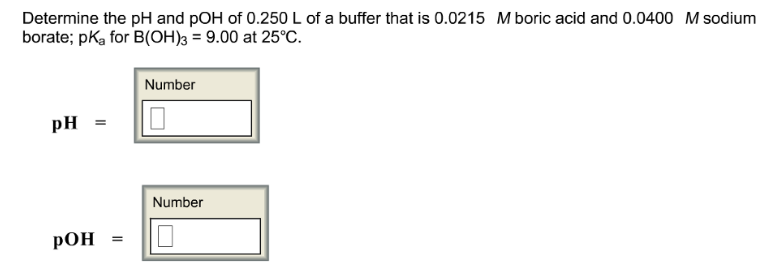# Problem: Determine the pH and pOH of 0.250 L of a buffer that is 0.0215 M boric acid and 0.0400 M sodium borate: pKa for B(OH)3 = 9.00 at 25°C. pH = pOH =

###### FREE Expert Solution
83% (231 ratings)###### Problem Details

Determine the pH and pOH of 0.250 L of a buffer that is 0.0215 M boric acid and 0.0400 M sodium borate: pKa for B(OH)3 = 9.00 at 25°C.

pH =

pOH =